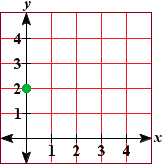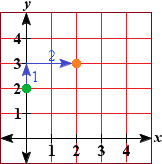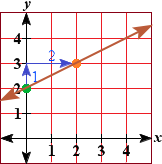Slope-Intercept Form

Slope-Intercept Form

An equation is in slope-intercept form, if it is in the form:

y = mx + b

The line will always be a straight line with a slope of m and a y-intercept of (0, b).

Example

Determine the slope and y-intercept of the following line:

-4x + 2y = 16

Solution

Write the original equation in slope-intercept form by adding 4x to each side and then dividing everything by two:

-4x + 2y + 4x = 16 + 4x

(2y) / 2 = (4x + 16) / 2

y = 2x + 8

Therefore the slope is 2 and the y-intercept is (0, 8), or 8.

You can also use the slope and y-intercept to graph a line.

Example

Graph the equation: y = 1/2x + 3

Solution

Using the slope-intercept form we see that the y-intercept is 3, so the first point is at (0, 3)The slope is 1/2, so we can plot a second point by moving up 1 unit and then to the right 2 units.Finally we draw a line through both points.